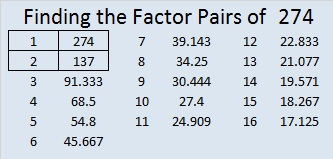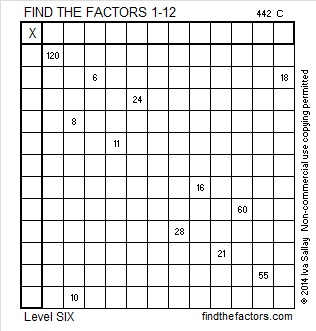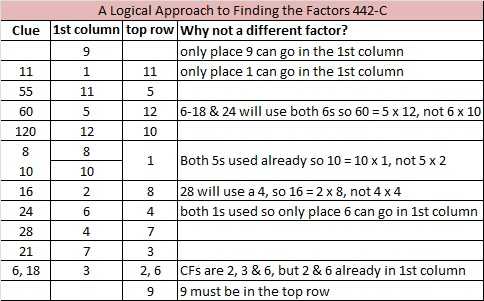# 274 and Level 6

• 274 is a composite number.
• Prime factorization: 274 = 2 x 137
• The exponents in the prime factorization are 1 and 1. Adding one to each and multiplying we get (1 + 1)(1 + 1) = 2 x 2 = 4. Therefore 274 has 4 factors.
• Factors of 274: 1, 2, 137, 274
• Factor pairs: 274 = 1 x 274 or 2 x 137
• 274 has no square factors that allow its square root to be simplified. √274 ≈ 16.553.I recommend using logic and not guess and check to solve the Find the Factors puzzles, but the logic for the one below can be a little tricky. If necessary, scroll down for one piece of reasoning that may be helpful if you get stuck in the middle of trying to solve this one.Print the puzzles or type the factors on this excel file: 12 Factors 2014-10-20

Notes on some logic needed for this puzzle:

• The choices for 24 are 2 x 12, 3 x 8, or 4 x 6.
• Because 28 = 4 x 7, 16 = 2 x 8, not 4 x 4. Thus 16 will use one of the 2’s. Either 6 or 8 will use the other 2, so we eliminate  2 x 12 = 24.
• Also 21 will use one of the 3’s while 6 or 18 will use the other. Thus we eliminate  3 x 8 = 24.
• Therefore 24 = 4 x 6 or 6 x 4.## 2 thoughts on “274 and Level 6”

1.Thalia

Hi there,
Could you pop me on your mailing list?
•ivasallay